# Difference between revisions of "0708-1300/Homework Assignment 1"

Jump to: navigation, search

Announcements go here

## Reading

Read sections 1-5 of chapter II of Bredon's book three times:

• First time as if you were reading a novel - quickly and without too much attention to detail, just to learn what the main keywords and concepts and goals are.
• Second time like you were studying for an exam on the subject - slowly and not skipping anything, verifying every little detail.
• And then a third time, again at a quicker pace, to remind yourself of the bigger picture all those little details are there to paint.

## Doing

• Solve and submit the following two problems:
1. Show explicitly that the restricted implicit function theorem, with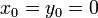$x_0=y_0=0$ and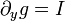$\partial_yg=I$, is equivalent to general implicit function theorem, in which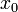$x_0$ and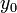$y_0$ are arbitrary and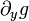$\partial_yg$ is an arbitrary invertible matrix.
2. Show that the definition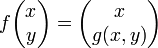$f\begin{pmatrix}x\\y\end{pmatrix}=\begin{pmatrix}x\\g(x,y)\end{pmatrix}$ reduces the implicit function theorem to the inverse function theorem. A key fact to verify is that differential of$f$ at the relevant point is invertible.
• Solve the following problems from Bredon's book, but submit only the solutions of the problems marked with an "S":
problems on page(s)
2, S3, S4, 5 71
1, S2 75-76
1-4 80

Important. Note the change from an earlier version of this assignment - Franklin Vera and Damir Kinzebulatov found that exercise 1 on page 71 of Bredon's book is wrong, so it was removed from the assignment and replaced by exercise 3.

## Due Date

This assignment is due in class on Thursday October 4, 2007.